# KSEEB SSLC Class 10 Maths Solutions Chapter 3 Pair of Linear Equations in Two Variables Ex 3.5

KSEEB SSLC Class 10 Maths Solutions Chapter 3 Pair of Linear Equations in Two Variables Ex 3.5 are part of KSEEB SSLC Class 10 Maths Solutions. Here we have given Karnataka SSLC Class 10 Maths Solutions Chapter 3 Pair of Linear Equations in Two Variables Exercise 3.5.

## Karnataka SSLC Class 10 Maths Solutions Chapter 3 Pair of Linear Equations in Two Variables Exercise 3.5

Question 1.
Which of the following pairs of linear equations has unique solution, no solution, or infinitely many solutions. In case there is a unique solution, find it by using cross multiplication method.
(i) x – 3y – 3 = 0
3x – 9y – 2 = 0
(ii) 2x + y = 5
3x + 2y = 8
(iii) 3x – 5y = 20
6x – 10y = 40
(iv) x – 3y – 7 = 0
3x – 3y – 15 = 0
Solution:
(i) x – 3y – 3 = 0 …………. (i)
3x – 9y – 2 = 0 ……………… (ii)
Here, a1 = 1, b1 = -3, c1 = -3
a2 = 3, b2 = -9, c2 = -2
$$\frac{\mathrm{a}_{1}}{\mathrm{a}_{2}}=\frac{1}{3} \quad \frac{\mathrm{b}_{1}}{\mathrm{b}_{2}}=\frac{-3}{-9}=\frac{1}{3}$$
$$\frac{c_{1}}{c_{2}}=\frac{-3}{-2}=\frac{3}{2}$$
Here, $$\frac{a_{1}}{a_{2}}=\frac{b_{1}}{b_{2}} \neq \frac{c_{2}}{c_{2}}$$
∴ No solution for these.

(ii) 2x + y = 5
3x + 2y = 8
2x + y – 5 = 0 → (1)
3x + 2y – 8 = 0 → (2)
Here, a1 = 2, b1 = 1, c1= – 5
a2 = 3, b2 = 2, c2 = – 8$\frac{a_{1}}{a_{2}}=\frac{2}{3} \quad \frac{b_{1}}{b_{2}}=\frac{1}{2} \quad \frac{c_{2}}{c_{2}}=\frac{-5}{-8}=\frac{5}{8}$
Here,$\frac{a_{1}}{a_{2}} \neq \frac{b_{1}}{b_{2}}$
∴ Many solutions are there for these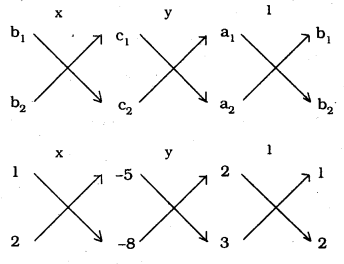$$\frac{\mathbf{x}}{1 x-8-(2 x-5)}=\frac{y}{-5 \times 3-(-8 \times 2)}=\frac{1}{2 \times 2-3 \times 1}$$
$$\frac{x}{-8+10}=\frac{y}{-15+16}=\frac{1}{4-3}$$
$$\frac{\mathbf{x}}{+2}=\frac{\mathbf{y}}{1}=\frac{1}{1}$$
$$\frac{\mathbf{x}}{+2}=\frac{1}{1} \quad \mathbf{x}=2$$
$$\frac{y}{1}=\frac{1}{1} \quad y=1$$
∴ Unique solution: x = 2, y = 1

(iii) 3x – 5y = 20
6x – 10y = 40
3x – 5y – 20 = 0 → (1)
6x – 10y – 40 = 0 → (2)
Here, a1 = 3 b1 = – 5, c1 = – 20
a2 = 6, b= – 10, c2 = – 40$\frac{\mathrm{a}_{1}}{\mathrm{a}_{2}}=\frac{3}{6}=\frac{1}{2} \quad \frac{\mathrm{b}_{1}}{\mathrm{b}_{2}}=\frac{-5}{-10}=\frac{1}{2}$$\frac{c_{1}}{c_{2}}=\frac{-20}{-30}=\frac{1}{2}$
Here,$\frac{\mathrm{a}_{1}}{\mathrm{a}_{2}}=\frac{\mathrm{b}_{1}}{\mathrm{b}_{2}}=\frac{\mathrm{c}_{1}}{\mathrm{c}_{2}}$
Infinite solutions are there for these.

(iv) x – 3y – 7 = 0 …………… (i)
3x – 3y – 15 = 0 …………….. (ii)
a1 = 1, b1 = -3, c1 = -7
a2 = 3, b2 = -3, c2 = -15
$$\frac{a_{1}}{a_{2}}=\frac{1}{3} \quad \frac{b_{1}}{b_{2}}=\frac{-3}{-3}=\frac{1}{1}$$
$$\frac{c_{1}}{c_{2}}=\frac{-7}{-15}=\frac{7}{15}$$
Here, $$\frac{\mathrm{a}_{1}}{\mathrm{a}_{2}} \neq \frac{\mathrm{b}_{1}}{\mathrm{b}_{2}}$$
∴ Many solutions are there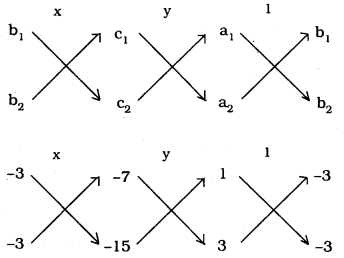$$\frac{x}{-3 x-15-(-3 x-7)}=\frac{y}{-7 \times 3-(-15 \times 1)}=\frac{1}{1 x-3-(3 x-3)}$$
$$\frac{x}{45-21}=\frac{y}{-21+15}=\frac{1}{-3+9}$$
$$\frac{x}{24}=\frac{y}{-6}=\frac{1}{6}$$
If $$\frac{x}{24}=\frac{1}{6}$$ then
6x = 24
$$x=\frac{24}{6}=4$$
If $$\frac{y}{-6}=\frac{1}{6}$$ then
6y = -6
$$y=\frac{-6}{6}$$
∴ y = -1
∴ Unique solutions are there for these x = 4, y = -1.

Question 2.
(i) For which values of a and b does the following pair of linear equations have an infinite number of solutions ?
2x + 3y = 7
(a – b) x + (a + b) y = 3a + b – 2
(ii) For which value of k will the following pair of linear equations have no solution ?
3x + y = 1
(2k – 1)x + (k – 1)y = 2k + 1
Solution:
(i) 2x + 3y = 7
2x + 3y – 7 = 0 ………… (i)
(a – b)x + (a + b)y = (3a + b – 2) ………… (ii)
(a – b)x + (a + b)y – (3a + b – 2) ……………. (ii)
Here, a1 =2, b1 = 3, c1 = -7
a2 = (a – b), b2 = (a + b), c2 = -(3a + b – 2)
If it has infinite solutions, then we have
$$\frac{\mathrm{a}_{1}}{\mathrm{a}_{2}}=\frac{\mathrm{b}_{1}}{\mathrm{b}_{2}}=\frac{\mathrm{c}_{1}}{\mathrm{c}_{2}}$$
$$\frac{2}{(a-b)}=\frac{3}{(a+b)}=\frac{-7}{-(3 a+b-2)}$$
If $$\frac{2}{(a-b)}=\frac{-7}{(-3 a-b+2)}$$ then
2(-3a – b + 2) = -7(a – b)
-6a – 2b + 4 = -7a + 7b
-6a + 7a – 2b – 7b = -4
a – 9b = 4 ………… (i)
If $$\frac{3}{(a+b)}=\frac{-7}{(-3 a-b+2)}$$ then
3(-3a – b + 2) = -7(a + b)
-9a- 3b + 6 = -7a – 7b
-9a + 7a – 3b + 7b = -6
-2a + 4b = -6
-a + 2b = -3 …………. (ii)
From eqn. (i) + eqn. (ii),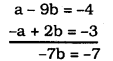7b = 7
$$b=\frac{7}{7}=1$$
Substituting the value of ‘b’ in eqn. (i),
a – 9b = -4
a – 9(1) = -4
a – 9 = -4
a = -4 + 9
a = 5
∴ a = 5, b= 1.

(ii) If the linear pair do not have solutions, then we have$\frac{a_{1}}{a_{2}}=\frac{b_{1}}{b_{2}} \neq \frac{c_{1}}{c_{2}}$
3x + y – 1 = 0 → (1)
(2k – 1)x + (k – 1)y – (2k + 1) = 0 → (2)
Here a1 = 3, b1 = 1, c1= 1
a2 = (2k – 1), b2 = (k – 1), c2 = -(2k + 1)$\frac{\mathbf{a}_{1}}{\mathbf{a}_{2}}=\frac{\mathbf{b}_{\mathbf{l}}}{\mathbf{b}_{2}}$$\frac{3}{(2 k-1)}=\frac{1}{(k-1)}$
3(k – 1) = 1(2k – 1)
3k – 3 = 2k – 2
3k – 2k = – 2 + 3
∴ k = 1.

Question 3.
Solve the following pair of linear equations by the substitution and cross-multiplication methods:
8x + 5y = 9
3x + 2y = 4
Solution:
8x + 5y = 9 ……………. (i)
3x + 2y = 4 …………….. (ii)
(1) Substitution Method:
8x + 5y = 9
8x = 9 – 5y
$$x=\frac{9-5 y}{8}$$
Substituting the value of ‘x’ in eqn (i)
3x + 2y = 4
$$3\left(\frac{9-5 y}{8}\right)+2 y=4$$
$$\frac{27-15 y}{8}+\frac{2 y}{1}=4$$
$$\frac{27-15 y+164}{8}=4$$
27 + y = 8 × 4
27 + y = 32
y = 32 – 27
∴ y = 5
Substituting the value of ‘y’ in
$$x=\frac{9-5 y}{8}$$
$$=\frac{9-5 \times 5}{8}$$
$$=\frac{9-25}{8}$$
$$=\frac{-16}{8}$$
∴ x = -2
∴ x = -2, y = 5
(2) Cross-Multiplication Method:
8x + 5y – 9 = 0 …………. (i)
3x + 2y – 4 = 0 …………. (ii)
Here, a1 = 8 b1 = 5 c1=-9
a2 = 3, b2 = 2, c2 = -4
8x + 5y = 9 3x + 2y = 4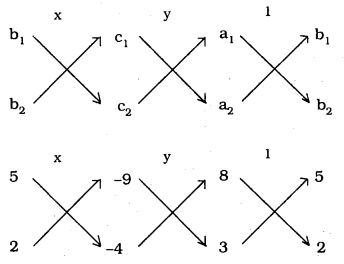$$\frac{x}{5 x-4-(2 x-9)}=\frac{y}{-9 \times 3-(-4 \times 8)}=\frac{1}{8 \times 2-3 \times 5}$$
$$\frac{x}{-20+18}=\frac{y}{-27+32}=\frac{1}{16-15}$$
$$\frac{\mathbf{x}}{-2}=\frac{y}{5}=\frac{1}{1}$$
$$\frac{\mathrm{x}}{-2}=1 \quad \mathrm{x}=-2$$
$$\frac{\mathrm{y}}{5}=1 \quad \mathrm{y}=5$$

Question 4.
Form the pair of linear equations in the following problems and find their solutions (if they exist) by any algebraic method :
(i) A part of monthly hostel charges is fixed and the remaining depends on the number of days one has taken food in the mess. When a student A takes food for 20 days she has to pay Rs. 1000 as hostel charges whereas a student B, who takes food for 26 days, pays Rs. 1180 as hostel charges. Find the fixed charges and the cost of food per day.
Solution:
Fixed hostel charges are Rs. x.
Amount to be paid to be Rs. y.
x + 20y = 1000 …………. (i)
x + 26y = 1180 …………. (ii)
By elimination method, we can find solution.
Subtracting eqn. (ii) from eqn. (i),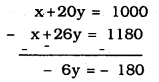– 6y =- 180
$$\mathbf{y}=\frac{180}{6}$$
∴ y = Rs. 30
Substituting the value of ‘y’ in eqn. (i),
x + 20y = 1000
x + 20(30) = 1000
x + 600 = 1000
∴ x = 1000 – 600
∴ x = Rs. 400
Fixed charge, x = Rs. 400
Amount to be paid, y = Rs. 30.

(ii) A fraction becomes $$\frac{1}{3}$$ when 1 is subtracted from the numerator and it becomes $$\frac{1}{4}$$ when 8 is added to its 4 denominator. Find the fraction.
Solution:
Let the fraction be$\frac{x}{y}$$\frac{x-1}{y}=\frac{1}{3}$
3(x – 1) = y
3x – 3 = y
3x – y – 3 = 0 → (1)$\frac{x}{y+8}=\frac{1}{4}$
4x = y + 8
4x – y – 8 = 0 → (2)
By Substitution method we can find out solution:
From eqn. (i),
3x – y – 3 = 0
y = 3x – 3
Substituting the value of y’ in eqn. (2),
4x – y – 8 = 0
4x – (3x – 3) – 8 = 0
4x – 3x + 3 – 8 = 0
x – 5 = 0
∴ x = 5.
Substituting the value of ‘x’ in
y = 3x – 3,
= 3(5) – 3
= 15 – 3
∴ y = 12
∴ x = 5, y = 12
∴ Fraction is$\frac{x}{y}=\frac{5}{12}$

(iii) Yash scored 40 marks in a test, getting 3 marks for each right answer and losing 1 mark for each wrong answer. Had 4 marks been awarded for each correct answer and 2 marks been deducted for each incorrect answer, then Yash would have scored 50 marks. How many questions were there in the test?
Solution:
Let the number of right answers be ‘x’, and the number of wrong answers be y’.
3x – y = 40 ……….. (i)
4x – 2y = 50 ………. (ii)
By Elimination method we can find our solution :
3x – y = 40 ……… (i)
2x – y = 25 ……….. (ii)
Subtracting eqn. (ii) from eqn. (i),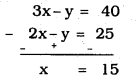Substituting the value of ‘x’ in eqn. (i),
3x – y = 40
3(15) – y = 40
45 – y = 40
-y = 40 – 45
-y = -5
∴ y = 5
Questions of right answers, x = 15
Questions of wrong answers, y = 5
∴ Total number of questions = x + y = 15 + 5 = 20

(iv) Places A and B are 100 km apart on a highway. One car starts from A and another from B at the same time. If the cars travel in the same direction at different speeds, they meet in 5 hours. If they travel towards each other, they meet in 1 hour. What are the speeds of the two cars ?
Solution:
Distance between places A and B is 100 km.
Speed of A car us ‘u’ km/hr.
Speed of B car is ‘v’ km/hr.
If the cars travel in the same direction at different speeds they meet in 5 hours about 20 km.
∴ u – v = 20 ………. (i)
If the cars travel in the same direction, total distance covered =100 km.
∴ u + v = 100 …….. (ii)
We can solve by Elimination method:
From eqn. (i) + eqn. (ii),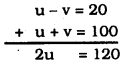$$u=\frac{120}{2}$$
∴ u = 60 km/hr.
Substituting the value of ‘u’ in Eqn. (ii),
u + v = 100
60 + v = 100
v = 100 – 60
v = 40 km/hr.
∴ u = 60 km/hr.
v = 40 km/hr.

(v) The area of a rectangle gets reduced by 9 square units, if its length is reduced by 5 units and breadth is increased by 3 units. If we increase the length by 3 units and the breadth by 2 units, the area increases by 67 square units. Find the dimensions of the rectangle.
Solution:
Length of rectangle is ‘x’ unit.
Breadth of rectangle is ‘y’ unit.
Area of rectangle = length × breadth = xy
(x – 5) (y + 3) = xy – 9
xy + 3x – 5y – 15 = xy – 9
3x – 5y = -9 + 15
3x – 5y = 6 …………. (i)
(x + 3) (y + 2) = xy + 67
xy + 2x + 3y + 6 = xy + 67
2x + 3y = 67 – 6
2x + 3y = 61 ……….. (ii)
Solving the equation by Elimination method :
Multiplying eqn. (i) by and eqn. (ii) by 5
9x – 15y = 18 ………….. (iii)
10x + 15y = 305 ………… (iv)
From eqn. (iii) + eqn. (iv)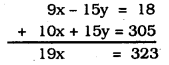$$x=\frac{323}{19}$$
∴ x = 17 unit.
Substituting the value of ‘x’ in eqn. (i),
3x – 5y = 6
3(17) – 5y = 6
51 – 5y = 6
-5y = 6 – 51
– 5y = – 45
5y = 45
$$y=\frac{45}{5}$$
∴ y = 9 units.
∴ Length of that rectangle, x = 17 unit